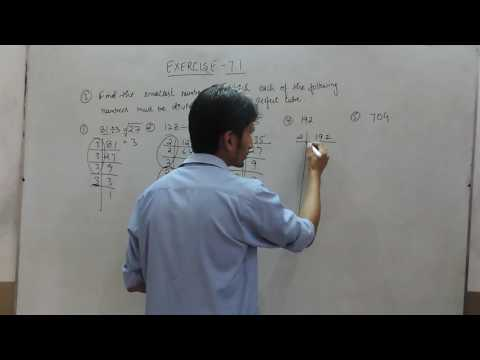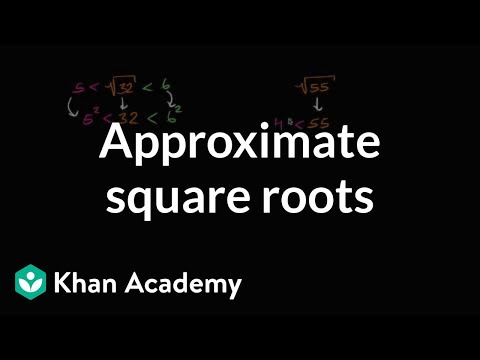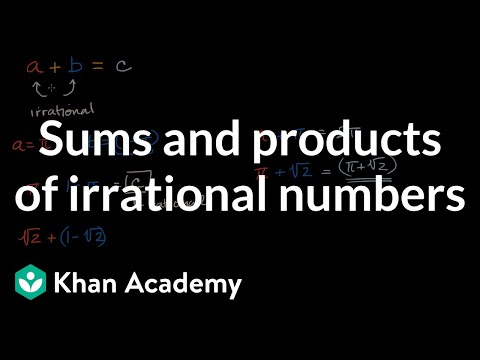# Exercise 7.1 - Question 3 NCERT/CBSE Solutions for Class 8th Maths Cubes and Cube Roots

##By TrueMaths

In this video we are solving class 8th NCERT - Chapter 7 Cubes and Cube Roots Exercise 7.2(Question-3).# Approximating square roots

##By Khan Academy

Learn how to find the approximate values of square roots. The examples used in this video are √32, √55, and √123. The technique used is to compare the squares of whole numbers to the number we're taking the square root of.# Cubes and Cube Roots - Maths - Class 8

##By LearnNext

If a and b are two natural numbers such that a3 = b, then b is called the cube of a. If the units digit of a3 is b, then the cubes of all numbers ending with a will have their units digit as b. The cubes of all numbers that end in 2 have 8 as the units digit. The cubes of all numbers that end in 3 have 7as the units digit.# Sums and products of irrational numbers

##By Khan Academy

Thinking about whether the sums and products of irrational numbers are rational or irrational.